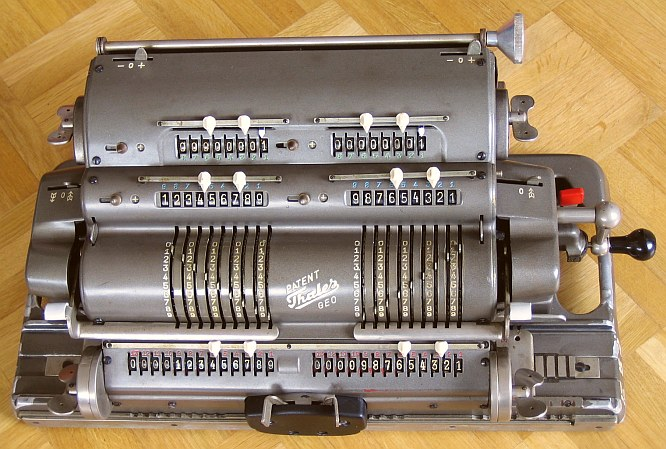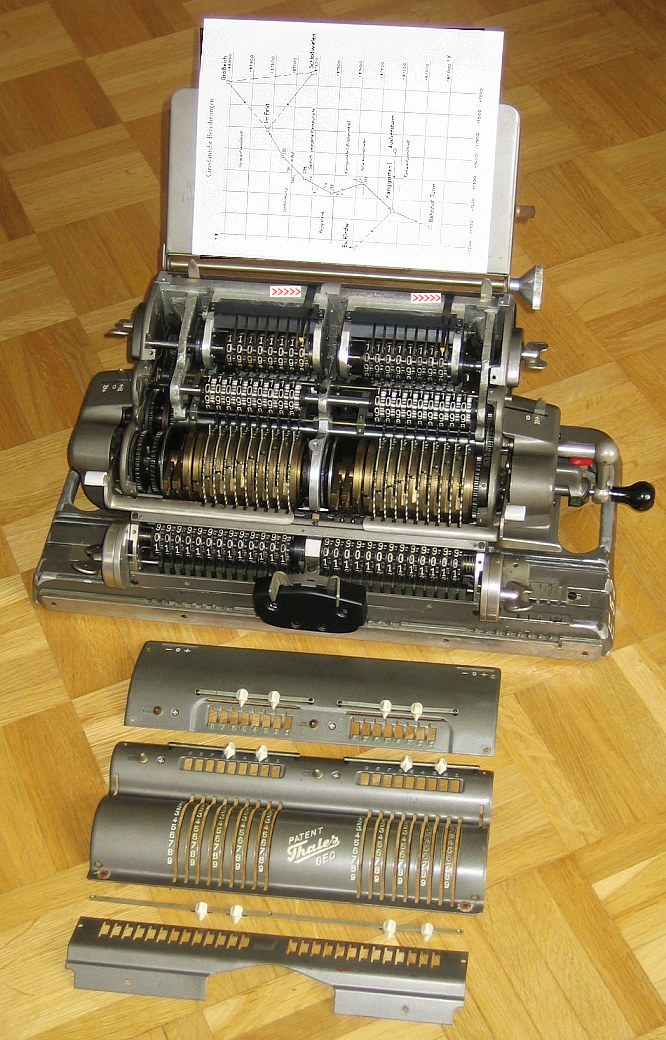previous <<==>> next

 THALES   GEO/RHave a look at the  THALES  MEZ ... Have a look at the  THALES  CER ... Have a look at the THALES »KA« ADDING MACHINES ...
 ```T E C H N I C A L D A T A of the Mechanical Calculator THALES »GEO/R« *************************************************************************** Serial Number: GEO 159884 ( right on base / front ) Dimensions: (ca.) Width = 16_1/2" / 42 cm Depth = 9 " / 23 cm Height = 6_1/2" / 17 cm Weight: (ca.) 31 lbs / 14 kg Mechanics: Pin-Wheel / Sprossenrad 10s Carry Mechanism Functions: Double-Calculator for Geo-Data Calculations Add, Subtract, Multiply, Divide Back-Transfer / Rueckuebertragung ( also cross-over !) Registers: CL/CR Counter = 2 x 8 Decimals (on Top) IL/IR Input = 2 x 9 Decimals AL/AR Arithmetic = 2 x 13 Decimals Manufacturer: THALES-WERK Rastatt / Baden Germany 1960 H O W T O U S E the THALES GEO/R **************************************** The DOUBLE CALCULATOR is symmetrical shaped. Each calculator works like the THALES-CER. The BACKTRANSFER-Lever (RED CAP) is located down behind crank. Initialized together with the associated (right/left) INPUT-CLEAR-LEVER and performed by turning the associated (right/left) ARITHMETIC-WING-NUT. Because the CARRIAGE is "shiftable through", it is possible to BACKTRANSFER the content of the RIGHT ARITHMETIC (AR) to the LEFT INPUT-UNIT (IL) or the content of the LEFT ARITHMETIC (AL) to the RIGHT INPUT-UNIT (IR). The DIRECTIONS OF COUNTING are settable independent: Equal, Non, Counter- directionional --- Also the way the ARITHMETIC-UNITS working ref. to the (left/right) MAIN-DIRECTION-LEVERs & CRANK (+/-) DIRECTION: Add./Sub. The COUNTERs & ARITHMETICs are pre-settable with affiliated toothed wheels. E X A M P L E : ( 987 - 6 )*54 - ( 321 + 9 )*54 = 35154 === === assume AL and AR are INTERMEDIATE RESULTS ... Pre-Set ARITHMETICS AL = 987 & AR = 321 Set INPUT-UNITS IL = 6 & IR = 9 Set LEFT MAIN-DIRECTION-LEVER to MINUS Set RIGHT MAIN-DIRECTION-LEVER to PLUS Set BOTH COUNTER-DIRECTIONS to PLUS Make ONE POSITIVE CRANK-Turn AL = 981 & AR = 330 B A C K T R A N S F E R AL -> IL & AR -> IR P R O C E D U R E : (.L.) LIFT LEFT CLEAR-INPUT-LEVER & PULL RED-CAP-LEVER RELEASE LEFT CLEAR-INPUT-LEVER Turn LEFT ARITHMETIC-WING-NUT for LEFT BACKTRANSFER ( RED-CAP-LEVER snaps back ) (.R.) LIFT RIGHT CLEAR-INPUT-LEVER & PULL RED-CAP-LEVER RELEASE RIGHT CLEAR-INPUT-LEVER Turn RIGHT ARITHMETIC-WING-NUT for RIGHT BACKTRANSFER ( RED-CAP-LEVER snaps back ) IL = 981 & IR = 330 AL = 0 & AR = 0 Set LEFT MAIN-DIRECTION-Lever back to PLUS Clear COUNTING-UNITS with its WING-NUTS CL = 0 & CR = 0 M U L T I P L I C A T I O N : Shift Carriage right to Position 2; Make 5 POSITIVE CRANKs Shift Carriage left to Position 1; Make 4 POSITIVE CRANKs Clear IR = 0 CL = 54 & CR = 54 AL = 52974 & AR = 17820 S U B T R A C T I O N : Shift Carriage so, that AR is under IL BACKTRANSFER AR -> IL IL = 17820 & IR = 0 Shift Carriage back to "normal" Position AL = 52974 & AR = 0 Make ONE NEGATIVE CRANK-Turn CL = 53 & CR = 53 AL = 35154 ===== Further INFO see ... http://www.rechnerlexikon.de/artikel/Thales_Geo Have a look at "Calculating Trickies" ... INSIDE VIEW: With the BIG KNOB right on top a PAPER-HOLDER is to flip up ... `````` ... thread-in the DUAL-POSITION-MARKERs before TOP-COVER replacement !!! ( see MARKS [ >>>>> ] & [ >>>>> ] ) impressum: *************************************************************************** © C.HAMANN http://public.BHT-Berlin.de/hamann 04/18/17 ```